Go beyond Einstein’s constant light speed!

Nimit Theeraleekul B. Eng. (Communication)

(28 Aug 2012, 1st revision 1 Feb 2013)

Abstract: “Constancy light speed referenced to any initial frame” is one of the basic assumptions in Einstein special theory of relativity; provided with a physical mechanism will change it from just an assumption to a real natural phenomenon. Then what we got from the improving is that we could understand physical mechanism of “relativistic effect” which gives rise to relativistic mechanics.

Indeed, improving physics theory by adding an appropriate mechanism is far-reaching; it could extend to Einstein general theory of relativity and quantum mechanical theory, which is then able to answer questions such as dark energy/matter, quantum entanglement, including Higgs. Finally it would pave the way to the theory of everything!

Introduction: Everyone who familiar with Einstein special theory of relativity (STR) knows that light speed is the same in any inertial frame, is one of the two of the theory basic foundations, and apparently it is likely to be so. Anyway, the problem is that it is just an assumption which is not so clearly be confirmed; or we may say that there is no good reason behind which could explain why and how it is be so!

Historically in nineteenth century physics, light wave was believed to move via luminiferous aether medium whose elastic properties are determined by Maxwell equations. Anyway the well known Michelson-Morley interference experiment result has interpreted that aether is not exist; this is the reason that Einstein proposed his constancy of light speed assumption and then STR was born.

1) How light travel? Nowadays mainstream physicists still believe that light wave travels (propagate) without using any medium to be carrier, i.e. it can travel by itself (via mutual creation between electric and magnetic field)! Anyway, the problem is that this seems impossible because both electric and magnetic field were created at the same time, and this will contradict to the principle of causality! 

Now let’s consider the Michelson-Morley interference experiment as mentioned. The crucial point of the experiment result is that whether there is an aether dragged by the earth or the interferometer armscontracted according to Einstein STR. Anyway, there is no experiment to date that directly confirms length contraction , but main physicists still believe in the contraction!

Anyway, recently there is a NASA report about the gravity probe experiment had proved that there is space-time dragging by the earth (according to general theory of relativity). So we could say that according to STR, the gravity probe experiment result has equivalently proved that there is aether dragged by the earth .

By the way, it is interesting to note that later Einstein has changed his idea , and admitted that there must be an unknown special kind – not the conventional aether which acts as the carrier of light wave, as was told by W. Pauli “…In this wider sense there does of course, exist an aether; only one has to bear in mind that it does not possess any mechanical properties…” .

2) Is vacuum space empty or not? Now here we will not refer to the Michelson-Morley experiment result; instead we will use a simple scientific experiment which will give an obvious result as below. And the experiment concept is a simple one; it is a two-solenoid experiment as show in the diag. fig.1. The key concept is the superposition of magnetic field B generated by a direct current flowing in a solenoid according to the formula,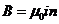[Where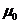is the permeability constant,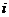is the current and n is number of turns.]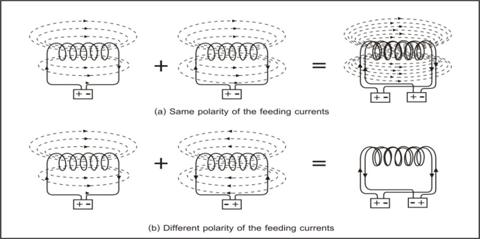Fig. 1. Two-solenoid experiment.

In diag. (a), sum of the created magnetic field is double, while in (b) the sum is zero. This is what it should be according to electromagnetic field theory, but the problem is where the created magnetic field energy was gone? We know that energy cannot disappear because it will violate the principle of conservation of energy! (Indeed if we could create energy from nothing or reverse, then we would be a great millionaire who could save the world energy’s crisis in the near future.)

So the only sensible answer is that the magnetic energy was used to cancel the opposite rotational stress in the physical medium within vacuum space! This is an obvious indirect prove for the existence of some physical things such as the aether, isn’t it?

3) What is vacuum medium? Someone may ask; are we going back to the old aether? The answer is yes and no! Yes, we are talking about some physical thing like the luminiferous aether; but no, it is not something which fills the empty vacuum space, instead it is vacuum space itself!

Our new concept is that vacuum medium is the infrastructure of our universe; we may say that the universe was created from vacuum medium energy! While the existing of vacuum medium is positive energy, its intrinsic gravity (i.e. internal contraction force energy which holds every infinisimal parts together) is negative energy. Then the conservation of the total energy of our universe was preserved!

In more detail, vacuum medium is a continuous isotopic homogenous medium which have a peculiar mechanical property. It is very thin constant mass density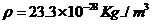(see section 7.1 in ) so it is permeable by all matters almost without any observed resistance! But it has very large elastic coefficient and sensitive to shear force while not to compressive force. The reason is because normally the elastic resistance against change of volume is larger than that change of relative orientation , especially if it is the volume of the whole universe’s space!

4) Origin of matters. After the universe was born and expanding its size from zero, at the same instance, every increasing parts of the volume (which was built with constant mass density of vacuum medium energy) has the tendency to collapse (due to their contraction force) and forming to be tiny black holes. Two sizes of the black hole (of vacuum medium) electrons and protons are the only two elementary particles created and floating in the sea of vacuum medium universe. (See Appendices A, the mechanism of electron black hole.)

Once electrons and protons are created, they are attracting each other forming to be Hydrogen atoms. When electron and proton were forced by external force (to overcome the tensile strength of the medium) to attach each other, then neutron particle is formed. Next when two protons were forced to attach with two neutrons, Helium nucleus was created, and so on. This is the basic steps of formation process of all natural matter in the universe! (See detail in Appendices B.)

5) Return to Maxwell electromagnetic field theory. Before going further, let’s talk some more about Maxwell theory which give rise to Maxwell equations (that was used in calculation for light wave); original the theory was derived from “granular model of space” which based on the aether concept . But nowadays the original model was ignored, modern physicists believe in field concept instead, so the theory using now has no mechanism behind!

Armed with vacuum medium concept, we can improve the theory by re-derivation the equations using an appropriate model via the mechanical properties of the vacuum medium. Then what we got from manipulating the equations is the light wave which is the manifest of the stress-strain relation of the medium, in which it was arisen from periodic vibration of the medium. Detail sees section 3&4 in .

Finally we could see that the improved theory is a better one, in which it could solve the existing unsolved problems in the conventional theory such as what the nature of electric and magnetic field are, what the Pointing vector is, etc. Detail sees section 5-12 in .

6) Vacuum medium based STR. Now we will summarize how to improve STR by using vacuum medium as the privileged reference frame (see detail in ). First we have to modify two basic hypothesis of STR i.e. to reinterpret concept of the conventional relativity principle to a new one; that is changing it from “laws of physics are the same reference to any inertial frame” to “laws of physics are the same reference to the vacuum medium privileged reference frame”!

Next we also have to change the second hypothesis of STR from “light velocity is the same for any inertial observer” to “light velocity is the same reference to the vacuum medium privileged reference frame”. (Actually we need not have to mention about this second hypothesis because the nature of light is also one of laws of physics!)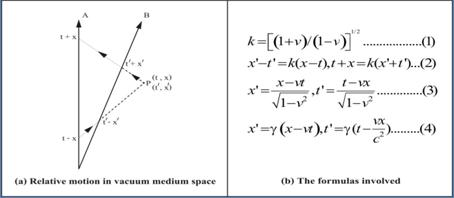Fig. 2. Lorentz transform. derivation based on vacuum medium space.

Finally we have to re-derive the Lorentz transformation according to the modified concepts, and we could found that actually it is easier than what was done in the conventional theory. By using the idea of k-calculus  as shown in fig.2 (a) and what we got is the Lorentz transformation as shown in fig.2 (b).

7) Physical meaning of relativistic effect. Once vacuum medium privileged reference frame was used, it is easy to understand the mechanism of all relativistic effect arisen in STR (in which conventionally it is just what we got from mathematical formula) as follow.

Starting with the Lorentz transformations derived by Lorentz himself . And according to the diag. in fig. 3 which we want to find the electric potential at point P (x, y, z) due to a moving charge q with constant speed v. If t = 0 is the moment when the charge q is at origin, and at the time t the charge is at x = vt, y = z = 0.  But actually the potential at point P arisen from the charge at B (not from A) at the moment it passes to A, which was called as the retarded time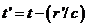From Lienard-Wiechert potential, the potential at P is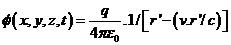And the potential in the present coordinate is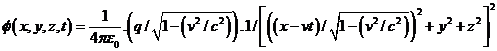…..(m).

If the charge is rest at the origin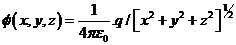……. (n)

Finally by compare the potential from the moving coordinate system (m) to the rest frame (n), it appear that the coordinate should be transformed by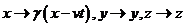[Where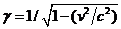]

This is Lorentz transformation which Lorentz discovered it. In summary, what we have done is transformed the coordinate of the potential generating point from retarded point A to the present point B. And the consequence is that the distance and time of the present position B, appeared to change their values according to the Lorentz transformation! This is the crucial point which will give rise to understand the mechanism of all relativistic effects, and to see more clearly we have to use the concept of vacuum medium space-time, next.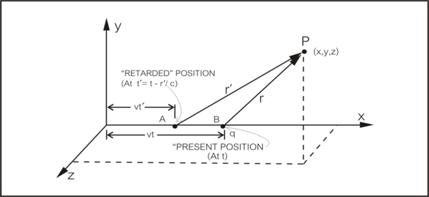Fig. 3. A moving charge’s potential with constant velocity.

8) Minkowskian space-time vs. Vacuum medium space-time. In contrary to Minkowskian space-time in the conventional STR (where the vacuum space is an empty space), vacuum medium space-time is a physical space as show in diag. fig.4, where a light source was placed at the origin O of the stationary absolute privileged vacuum medium referenced frame S, at time t = x = y = z = 0. Then light signal propagates radial outward as a sphere as,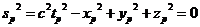Next, let us place the light source at the origin O’ of a moving frame S’ (referenced to vacuum medium rest frame). When O’ is at O and at t = t’ = 0 (while light source is start emitting), S’ is also moving with speed v (less than c). And when S’ is passing P’ toward Q, the emitting signal from P’ will reach P at the same instant that S’ is at Q.

By comparing the situation of the moving light source here with the moving charge in section 7, we could see that it is indeed equivalent!  Now if we consider only for the co-ordinate x, then the position P’ (fig.4) is the same position as A (the retarded time) in fig. 3. The reason behind is because the speed electric potential propagate is the same as speed of light.

In the same way as was done with the moving charge, if we transform the coordinate of the moving light source at P’ to the present position Q, then what we got is the distance and time of the present position Q (of the moving light source) appeared to change their values according to the Lorentz transformation!

Now let us compare Einstein derivation’s condition (light speed is constant referenced to the moving inertial frame) to our condition which we have done here (light speed is constant referenced to the absolute privileged vacuum medium frame); then what he got is the same Lorentz transformation. Also we could see that Einstein’s view that light speed is constant referenced to the moving inertial frame is the same as our view for the retarded position P’ in which it acting as moving inertial frame (that emit light with constant speed c)!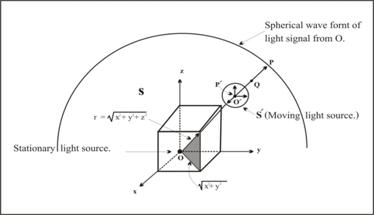Fig. 4. Vacuum medium space-time.

In conclusion we can say that the relativistic effects which arisen from relative motion (which was shown by Lorentz transformation) are because of the idea that the moving light source emits light from its present location, while actually its action occurs at early (retarded) time. This is the reason for length contraction and time dilation (as appeared in Lorentz transformation formulas) which are just a mathematical manipulation - not the true physical effects

9) Mechanism of “increasing mass”. Up to now someone may question - how about the evidences of the increasing mass and time dilation effects, are they real physical effects? The answer is yes, but they arisen from other cause of the relativistic effect! It is the effect arisen from resistive reaction of vacuum medium. Here is the summary which explains how the mechanism of the increasing mass works, first, as follow.

Return to section 7 which we have compared the potential in (m) with (n), now consider the term with charge q in the bracket. Then we will found that for the moving charge case in (m), the charge come with gamma factor which could be interpreted that the value of the charge is increasing by a factor of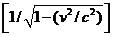.

Now we know that gravity has the same attraction rule as Coulomb law in electricity, if we replace the charge q with a mass then we would found that its gravitation potential is the same form as electric potential above. So the “moving” gravitational potential of the moving mass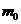at P is (m’) and has the same form as (m) below.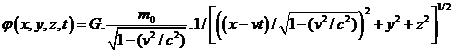….. (m’).

In the same way as (n), the “static” gravitational potential of a stationary massis (n’),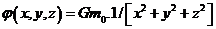….. (n’).

Finally by compare the potential in (m) with (n), now consider the term with massin the bracket. Then we will found that for the moving massin (m), come with gamma factor which could be interpreted that the value of the mass is increasing by a factor of. The reason behind for the “additional mass” is due to the “resistive action of vacuum medium” to the moving mass as mentioned early.

Now to explain about the conventional time dilation for a moving atomic clock, which was misinterpreted as a true time dilation; actually it is the slowing of the mechanism of the clock due to the resistive reaction of vacuum medium, in which it action slows the vibration of the atoms used as time reference in atomic clock (see detail in reference ).

10) Relativistic mechanics vs. Vacuum mechanics. Now we will show in more detail how the interpretation of conventional relativistic effect different from our new interpretation. First let us start with classical mechanics in which we know that Newton law is the same via Galilean transformation. And its main point is that mass of an object is constant whether it is moving or not!

Actually, what we have talked before about the increasing mass of a moving object, it is belongs to the field of relativistic mechanics which follow from STR. But the problem is that the conventional concept of STR, i.e. mass increase with velocity, is just an assumption! Anyway after improving the STR and we have found that the mechanism of the increasing mass is the resistance of vacuum medium, so it is more appropriate to recall the conventional relativistic mechanics as “Vacuum mechanics”.

Now let us consider a simple case of collision of point masses which shows in diag. fig.5 below. Where in (a) the situation before the collision in the reference frame S, (b) after the collision in S, (c) the situation before the collision in the reference frame S’ and (d) after the collision in S’.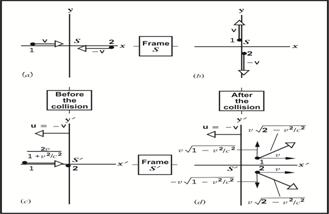Fig. 5. Two particles collision.

First by using the classical mechanics’ concept, the components of the momentum of the two-particle system in S are

Initial;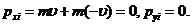Final;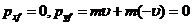. Thus the momentum is conserved!

Next, when viewing the same collision from the S’ frame which move relative to S with speed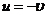. Together with using Lorentz velocity transformation for x’ and y’ components observed by S’ that are shown in (c) and (d), the momentum of the system are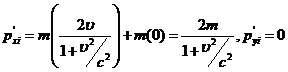,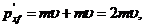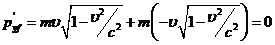Then the momentum is not conserved.          But if we use the relativistic formulas of momentum,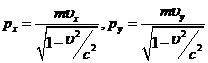Then… (p). And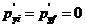. So the momentum is conserved.

Finally, to see Vacuum mechanics’ view, let’s rewrite equation (p) as below.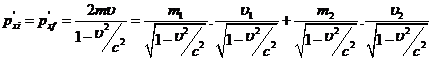…. (q)

(Where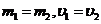.)

Looking at the right hand side, the first and third terms are what were called relativistic masses which are the increased masses that subjected to the resistance of vacuum medium as mentioned.  While the second and forth terms are called relativistic speeds which are the relative speed of both the relative moving masses.

Finally, we are ready to interpret the meaning of the three kind mechanics mentioned by compare them as in the table, together with referenced to equation (q). And remember that light speed formula is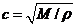(Where M = elasticity, ρ = mass density of medium).

 Type Vacuum space Light speed Mass increase Cause Classical mechanics empty ∞ No Nothing Relativistic mechanics empty c Yes Nothing Vacuum mechanics vacuum medium c Yes Resistive action

First, according to classical mechanics, because space is empty which mean ρ = 0, so we could say that c =. So the moving masses are constant because there is nothing to oppose their motion. This is corresponds to the concept of Galilean transformation in which Newton law is the same under its transformation!

Secondly, consider the conventional relativistic mechanics which is indeed very strange because its assumption is based on empty space while speed of light is still being c! Also while there is nothing to resist the moving masses, but the moving masses still increasing their mass (as if they were subject to some resistance)!

Thirdly, according to the Vacuum mechanics’ case, because space is not empty, so light speed is c (speed of light propagation in the medium ρ). Also the moving masses were subjected to the resistance of vacuum medium as mentioned early; this is a reasonable cause which make the masses appeared as if they are increased!

In conclusion it is reasonable and more realistic to say that when a mass moving at high speed (comparable to c), its mass appeared to increase due to the resistive reaction of vacuum medium according to relativistic mechanics (which actually it is vacuum mechanics). While at low speed (in daily life) the resistive reaction is negligible (gamma factor approach one), this is the concept of classical mechanics.

11) Could we catch up light wave? According to Einstein special theory of relativity in which light speed was assumed to be the fastest nature’s speed (i.e. nothing can move faster than light) and indeed it was confirmed in laboratory test. So as show in the figure, such a light wave riding (the same way as water wave riding) is not possible because light wave is too fast to catch up!

Unfortunately there is no explanation why it is be so, how does it work, or what is it mechanism? Does it mean that no mechanism is required? Certainly not, this is physics – not a magic! Here we will find it out together scientifically.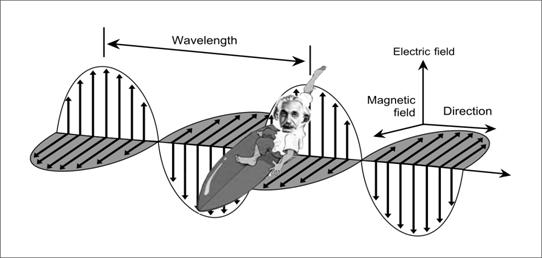Fig.6. Light wave riding is not possible – Einstein.

Now in classical mechanics ream, let us consider in detail about the behavior of a moving object’s speed (u) in the moving inertial frame (v) which was seen by an observer at rest frame as the composite speed w =u+v. Theoretically there is no speed limit about speed u and v, even for example w = 0.5c + 0.6c = 1.1c (where c = light speed) which imply that the moving object’s speed was observed as greater speed than light!

Next according to the relativistic formula in STR, the composite speed,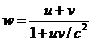.

At low speed of u and v compare to c, the denominator is approaching 1, then w =u+v return to the classical non-relativistic formula as mentioned above. For high speed of u such as u=c, we would found that w=c, no matter how much the speed of v. This means that the moving object’s speed was observed must be no greater speed than light!

Anyway, we know that the relativistic formula for composite speed was derived from the concept of space-time structure which based on light speed c. So there is no physical mechanism which explains how it works! Someone may argue that it is because the fast moving object will increase its mass toward infinity because the factor(as explain in section 9) when its moving speed approach light speed.

Again there is still no physical mechanism which explains how the increasing mass works, except the mechanism of vacuum medium – vacuum mechanics (as we have discussed in detail early). So we may conclude that it is impossible for light wave riding due to the resistive action of vacuum medium which retard the fast moving “surfer” on the light wave sea!

12) Vacuum mechanics a new way to theory of everything. Up to now we have shown how “mechanism of vacuum medium” could improve STR to be a more complete theory. Indeed, the benefit from improving physics theory by adding an appropriate mechanism – vacuum mechanics is far-reaching; it could extend to Einstein general theory of relativity, which then able to answer the unsolved questions such as dark energy/matter, the origin of matters and the mechanism of gravity (see detail in ).

Here let’s see dark energy/matter as an example, actually dark energy is vacuum medium energy which acts as the structural framework of our huge universe, so it is a great part of the total energy. While for dark matter, actually it is the manifest of rotational stress of vacuum medium which occurred because dragging force of the rotating galaxy.

Beside relativity theory, we could also use vacuum mechanics to improve quantum mechanical theory. Then what we got is a more complete theory which could explain some unsolved problem such as what the wave–particle duality is, or how quantum entanglement works, etc. Again armed with vacuum mechanics, it is not difficult to see that actually quantum particle’s wave is a co-moving wave created by the moving particle. And we could also see that actually quantum entanglement works via a quantum vacuum mechanics’ hidden variable! Detail sees in .

Another hot problem in quantum field theory is the mysterious Higgs of standard model of particle physics, which based on the concept that there is infinite creation and annihilation of virtual particle-antiparticle pairs (which came with an inescapable problem of infinite amount of vacuum energy in vacuum space ).  Again armed with vacuum mechanics, it is more reasonable that actually Higgs field is vacuum medium; while Higgs boson is disturbed part of vacuum medium occurred during the collision! Detail sees section 7.4 of .

Finally it is possible to use vacuum medium as the missing link to “connect relativity to quantum mechanics”, which then could guiding us toward the theory of everything. The reason is because all natural phenomena are the appearance of vacuum mechanics . Indeed we have no need for something which is beyond our perception such as an extra dimensions or universes, in order to reach the theory of everything; all we have done is just look for something around within our universe!

Conclusion & discussion. Up to now we have finished talking about what which mentioned in the “Abstract”, here are some additional comments.

1) What we have done so far, is trying to put some appropriate mechanisms in to our modern physics theories and then makes it possible to improve them to be more complete theories which could explain how the related real natural phenomena work.

2) To work with some physical mechanism, first we have to choose the appropriate related physical material, and here what we have used is vacuum medium. Fortunately, vacuum medium could be used as the ingredient for the mechanism in all modern physics theories.

3) As we have seen in this paper, vacuum medium seems to be the only ingredient for all natural phenomena; starting from being the infrastructure of vacuum space, all the matters existed in our universe including all of us who try to ask – who we are, why we are here and where we are going.

4) Everyone who familiar with the topic of this paper would known that it has a lot of things to say, anyway what we have talked about is just the starting point, and if we are right, it would pave the way to a great revolution in physics theory.

5) Finally we could found that this paper is one which not fully mathematical rigor. Anyway, it is not a toy theory; what we have done is just enough to give new ideas to the readers with some confidences. So what was left here should be the work of professionals who are involved in the matter to complete it for the virtue of academic merit and the progress of our world’s knowledge!

……………………………………..

Appendices

A) Electron (proton) - tiny black holes. This is the consequent hypothesis of vacuum medium which was inspired from the concept “matter is the condensed of energy” and Einstein’s electron theory [5’]. Here is the summary of section 3.2 in .

According to the Einstein’s theory, material masses of electrical elementary particles are held together by gravitational forces. Anyway, it faced with difficulty that ordinary gravitational force is too weak to do the work. Armed with vacuum mechanics’ concept, i.e. electron is a tiny black hole (formed by gravitational force of vacuum medium itself), then the difficult was eliminated.

In summary, by follow Einstein idea which based on the assumption of a material energy-momentum tensor in the present of gravitational fields, together with Maxwell’s energy tensor. Then what he had got is,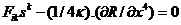…. (1).

The first term in equa. (1) is the Coulomb repulsive force which is held in equilibrium by a gravitational force in the second term.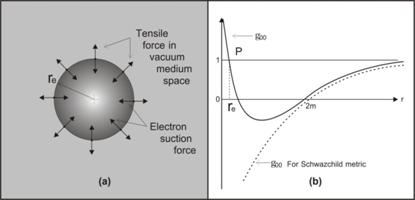Fig. 6. Electron black hole model.

By using the Reissner-Nordstrom solution, equa. (2), together with the concept that electron is a tiny black hole immerged in the surrounding of vacuum medium, then we would found that Reissner-Nordstrom solution should be a rational model of electron which could staying stable.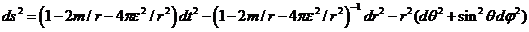…. (2).

[Where the second term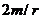in parenthesis is the gravitation field due to electron mass, and the third term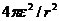is the gravitation field due to electron electric field.]

In fig.6 (a), it shows an electron sphere with radius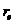surrounding by vacuum medium. On the surface of the sphere, the suction force of electron black hole was countered by the internal tensile force (stress) in the medium around, so the total force on the surface of the sphere is zero! In this situation theterm must equal to theterm, or equivalently we may say that the effect of curved space due to gravity energy was canceled by the effect due to electric energy. And then the line element metric will be Minkowski (flat) space-time there!

Now according to fig.6 (b), which is the graph between the metric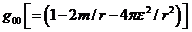and the radius r [11’], and we would found that at point P where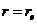,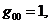then the calculated electron’s radiusis equal toIndeed, we found that it is the same order as the classical electron radius, so it give us confidence in the proposed electron black hole model!

B) Formation process of matters. Here is the summary which explained in section 3.3 of  and section 2 .

According to fig.7, here how matter was created, when proton and electron come close together, they will attract each other, forming to be hydrogen atom shown in (a). But they cannot attach together because of the existing of vacuum medium between! Anyway, if they were forced to attach together and tearing off the vacuum medium between, then a neutron particle will be created as shown in (b). And the resultant is the “attach force” between different size particles (which was dominant after the compressing force had overcome the tensile force of vacuum medium) was named as “weak force”!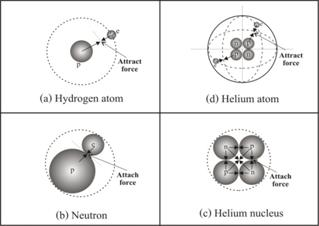Fig. 7. Formation process of matters.

Next if two neutrons and two protons were forced to attach together, then a nucleus of helium atom will be created as shown in (c). And the resultant is another “attach force” between two same size particles (which was dominant after the compressing force had overcome the tensile force of vacuum medium), and was named as “strong force”!

Finally, when the nucleus of helium atom was created, it will attract the nearby electrons and forming to be helium atom. This is the initial state of the process for forming all kind of the matters in our universe by using two elementary particles i.e. proton and electron!

By the way, to give us some confidence for the concept of charges particle are tiny black holes, we will use it to explain charge particles’ interaction. Conventionally “different type of charge particles attract while the same types repel” could explain by vacuum medium’s mechanism. Because any size of black hole attract each other, and also attract the surrounding medium, so under certain distance apart, the same sizes of tiny black hole repel each other, while different size attract. So a better concept is different size of charge particles attract while the same size repels. See detail in section 11 of .

References

 Oleg D. Jefimenko, “Causality Electromagnetic Induction and Gravitation” (Electret Scientific Company) 2nd Edition p.13-16.

 Richard A. Mould “Basic Relativity” (Springer-Verlag NY) Corrected 2nd printing 1995 p.82.

 http://phys.org/news/2011-05-gravity-probe-einstein-theories.html

 Frank Wilczek, “The Lightness of Being” (Basic Books) p. 82.

 W. Pauli, “Theory of Relativity” (Dover Publications) p.4. For [5’] p. 202-205.

 Nimit Theeraleekul,”Completed Einstein general theory of relativity (CGTR)” in author’s web.-

 Arnold Sommerfeld, “Mechanics of Deformable Bodies” (Academic Press) p. 107.

 James Blake Westgard, “Electrodynamics: A Concise Introduction” (Springer-Verlag NY) p. 90-95.

 Nimit Theeraleekul,”Completed Maxwell electromagnetic field theory (CEMT)” in author’s web.

 Nimit Theeraleekul,”Completed Einstein special theory of relativity (CSTR)” in author’s web.

 Ray d’Inverno “Introducing Einstein’s Relativity” (Oxford Uni. Press) Reprinted 1993 p. 25. For

[11’] p. 239-243.

 Richard P. Feynman “The Feynman Lecture on Physics” (Addison -Westley Pub.) Volume 2

p. 21-12 – 21-13.

 Nimit Theeraleekul,”What really the “time dilation” in relativity means?” in author’s web.

 Halliday, R. Resnick, and K. Kraneρ, “Physics” (John Wiley & Sons) Vol. 1 Extended Version 4th

Edition, p.482 - 483.

 Nimit Theeraleekul,”Completed quantum mechanical theory (CQMT)” in author’s web.

 Peter W. Milonni,”The Quantum Vacuum” (Academic Press), p. 48-49.

 Nimit Theeraleekul, “Vacuum mechanics a realistic theory of everything” in author’s web.

 Nimit Theeraleekul, “Vacuum mechanics a new approach to theory of everything” in author’s web.

…………………………………